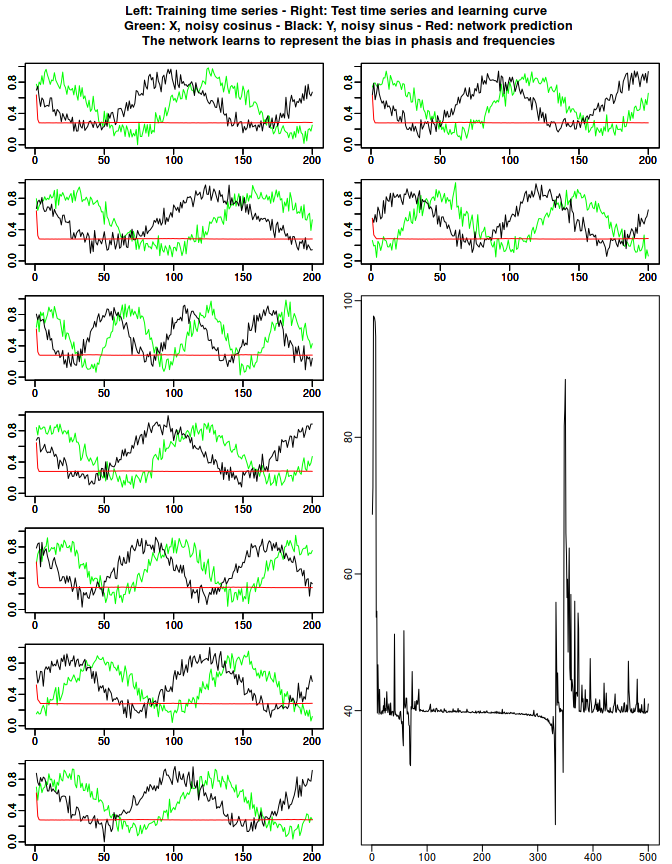Package

The package is loaded using:

library(rnn)

Generate data.

# synthetic time serie prediction
sample_dim <- 9
time_dim <- 200
X <- data.frame()
Y <- data.frame()

# Genereate a bias in phase
bias_phase <- rnorm(sample_dim)
# Generate a bias in frequency
bias_frequency = runif(sample_dim,min=5,max=25)

# Generate the noisy time series, cosinus for X and sinus for Y, with a random bias in phase and in frequency
for(i in seq(sample_dim)){
X <- rbind(X,sin(seq(time_dim)/bias_frequency[i]+bias_phase[i])+rnorm(time_dim,mean=0,sd=0.2))
Y <- rbind(Y,cos(seq(time_dim)/bias_frequency[i]+bias_phase[i])+rnorm(time_dim,mean=0,sd=0.2))
}
X <- as.matrix(X)
Y <- as.matrix(Y)

# Normalize between 0 and 1 for the sigmoid
X <- (X-min(X))/(max(X)-min(X))
Y <- (Y-min(Y))/(max(Y)-min(Y))

Train the model.

# Train with all but the 2 lasts sample
model <- trainr(Y = Y[seq(sample_dim-2),],X = X[seq(sample_dim-2),],learningrate = 0.05,hidden_dim = c(16),numepochs=500,batch_size = 1,momentum = 0,learningrate_decay = 1)

Plot it using testing data.

# Plot and predict all samples
layout(cbind(seq(sample_dim-2),c((sample_dim-1):sample_dim,rep(sample_dim+1,sample_dim-4))))
par(mar=c(1.5,2,1,1),xaxt="s",yaxt="s",mgp=c(1.5,0.5,0),oma=c(0,0,4,0))
for(i in seq(sample_dim)){
plot(X[i,],type="l",col="green",ylim=c(0,1),xlab="",ylab="")
par(new=T)
plot(Y[i,],type="l",ylim=c(0,1),xlab="",ylab="")
par(new=T)
plot(predictr(model,X)[i,],type="l",ylim=c(0,1),col="red",xlab="",ylab="")
}
plot(colMeans(model\$error),type="l",xlab="",ylab="",xlim=c(1,500))
title(main="Left: Training time series - Right: Test time series and learning curve
Green: X, noisy cosinus - Black: Y, noisy sinus - Red: network prediction
The network learns to represent the bias in phasis and frequencies",outer=T)